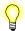# qdb_stmt_exec()

Execute a precompiled statement

## Synopsis:

```#include <qdb/qdb.h>

int qdb_stmt_exec( qdb_hdl_t *hdl,
int stmtid,
qdb_binding_t *bindings,
uint8_t binding_count );```

## Arguments:

hdl
A pointer to the database handle.
stmtid
The ID of a pre-compiled statement, returned by qdb_stmt_init().
bindings
An array of qdb_binding_t structures filled in with pointers to data that will be bound in to the variable parameters in the pre-compiled statement. See below.
binding_count
The number of items in bindings.

qdb

## Description:

This function executes a precompiled statement that was previously prepared with qdb_stmt_init().

### The qdb_binding_t structure

The qdb_binding_t structure has at least these members:

int index
The index of the variable parameter in the precompiled statement that this data should be bound to. The placeholder is in the form of ?n, where n is a number between 1 and 999.
int type
The type of the data. Can be one of: QDB_NULL, QDB_BLOB, QDB_TEXT, QDB_INTEGER, or QDB_REAL.
int len
The length of the data argument. This number should exclude '\0' for QDB_TEXT, should be sizeof(uint64_t) for QDB_INTEGER and sizeof(double) for QDB_REAL.
void *data
A pointer to the data to be bound in.

You can initialize an instance of qdb_binding_t using one of the convenience macros below. In the macro prototypes, bind is the address of the qdb_binding_t structure, i is the index member, t is the type member, l is the len member, and d is the data:

QDB_SETBIND(bind, i, t, l, d)
Bind in any type of data.
QDB_SETBIND_INT(bind, i, d)
Bind in an integer.
QDB_SETBIND_NULLbind, i)
Bind in NULL.
QDB_SETBIND_TEXT(bind, i, d)
Bind in text.There is a limit to the amount of data that can be sent to a database when using qdb_stmt_exec(). This limit is the lesser of the following values: the limits set by the database x = 231 - ( binding_count + 1 ) × 12, where x is the data limit, in bytes

## Returns:

>0
Success.
-1
An error occurred (errno is set).

## Examples:

See qdb_stmt_init().

## Classification:

QNX Neutrino

Safety:
Interrupt handler No
Signal handler No